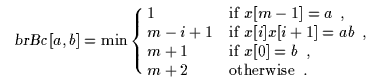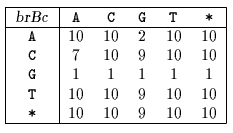Next: Smith algorithm Up: ESMAJ Previous: Zhu-Takaoka

# Berry-Ravindran algorithm

Main features
• hybrid of the Quick Search and Zhu and Takaoka algorithms;
• preprocessing phase in O(m+2) space and time complexity;
• searching phase in O(mn) time complexity.
Description

Berry and Ravindran designed an algorithm which performs the shifts by considering the bad-character shift (see chapter Boyer-Moore algorithm) for the two consecutive text characters immediately to the right of the window.

The preprocessing phase of the algorithm consists in computing for each pair of characters (a, b) with a, b inthe rightmost occurrence of ab in axb. For a, b inThe preprocessing phase is in O(m+2) space and time complexity.

After an attempt where the window is positioned on the text factor y[j .. j+m-1] a shift of length brBc[y[j+m],y[j+m+1]] is performed. The text character y[n] is equal to the null character and y[n+1] is set to this null character in order to be able to compute the last shifts of the algorithm.

The searching phase of the Berry-Ravindran algorithm has a O(mn) time complexity.

The C code
```void preBrBc(char *x, int m, int brBc[ASIZE][ASIZE]) {
int a, b, i;

for (a = 0; a < ASIZE; ++a)
for (b = 0; b < ASIZE; ++b)
brBc[a][b] = m + 2;
for (a = 0; a < ASIZE; ++a)
brBc[a][x] = m + 1;
for (i = 0; i < m - 1; ++i)
brBc[x[i]][x[i + 1]] = m - i;
for (a = 0; a < ASIZE; ++a)
brBc[x[m - 1]][a] = 1;
}

void BR(char *x, int m, char *y, int n) {
int j, brBc[ASIZE][ASIZE];

/* Preprocessing */
preBrBc(x, m, brBc);

/* Searching */
y[n + 1] = '\0';
j = 0;
while (j <= n - m) {
if (memcmp(x, y + j, m) == 0)
OUTPUT(j);
j += brBc[y[j + m]][y[j + m + 1]];
}
}

```
The example

Preprocessing phaseThe star (*) represents any character in\{A, C, G, T}.
brBc table used by Berry-Ravindran algorithm.

Searching phase

References
• BERRY, T., RAVINDRAN, S., 1999, A fast string matching algorithm and experimental results, in Proceedings of the Prague Stringology Club Workshop`99, J. Holub and M. Simánek ed., Collaborative Report DC-99-05, Czech Technical University, Prague, Czech Republic, 1999, pp 16-26.Next: Smith algorithm Up: ESMAJ Previous: Zhu-Takaoka

e-mails: {Christian.Charras, Thierry.Lecroq }@laposte.net
Tue Jan 14 15:03:31 MET 1997